# Articles

The Mathematics category serves as a comprehensive guide for both parents and students navigating the complexities of math education. Here, you'll find a wide array of articles that cover foundational to advanced topics, offering clarity and insight that can aid in both classroom learning and homework sessions. Whether you're seeking to understand the basics or aiming for higher-level mastery, this section provides a diverse set of tools to meet your needs.### 10 Interesting Careers in Math and Science

There are math careers and science careers, and there are also lots of occupations that incorporate both subjects. Many of these careers fall into the STEM category: science, technology, engineering, and mathematics. STEM jobs are available in the government as well as the private sector.### States That Spend the Most on Education

Investing in the future of our children via their education can help them build the lives they want. But education comes at a price—and a high one, at that. And some states invest more in their students than others. Find out which states have the highest spending per student!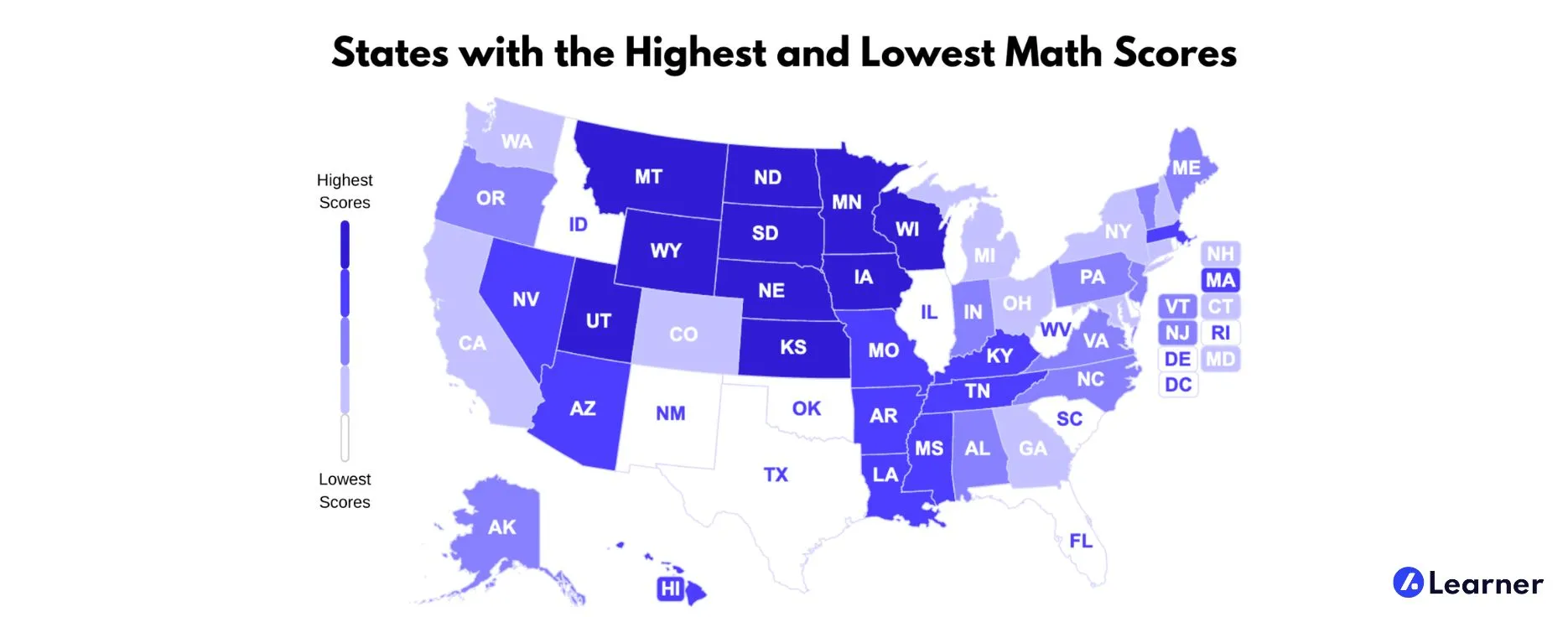### States with the Highest and Lowest Math Scores

At Learner, we understand both the difficulty and the necessity of math as a subject and wanted to know what math looks like in the United States. We took a look at the average SAT math scores throughout the nation to find which states had the highest and the lowest. Find out how your state stacks up against the rest!### How Can I Score Well on the GED® Math Test?

Passing the General Education Development® (GED®) test gives a person the equivalent of a high school diploma. The math test is one of five sections of the exam. To score well on the GED® math test, you need a sound understanding of the concepts tested in each of the four content areas covered in this section.### What Is Interdisciplinary Mathematics?

Interdisciplinary mathematics is a field of mathematics that merges math expertise with proficiency in another discipline, usually science, business, medicine or engineering. This contemporary training approach to educating math majors caters to employers who need professionals with strong math skills but who have a broader scope of application than traditional mathematics majors.### How Do I Score Well on ASVAB Math?

The Armed Services Vocational Aptitude Battery (ASVAB) is given to enlistees to assess their mental capabilities and to determine what kind of military jobs and training they will receive by the U.S. Armed Services. There are two ASVAB math subtests: Arithmetic Reasoning and Mathematics Knowledge.### What are Math Worksheets?

Math worksheets are meant to provide extra skills practice, whether the topic is addition or calculus. While worksheets often contain columns of problems to solve, sometimes they present their information in a more entertaining manner, such as finding the answers to fill in a crossword or other puzzle.### What Are the Different Types of Math Internships?

Mathematics majors can pursue one of a number of career paths. Obtaining math internships is a way that students can learn what this field has to offer in terms of opportunities. Industries such as aerospace, technology, and finance are all categories of employment where math internships can be uncovered.### What is Interactive Math?

Interactive math is a method by which a person can learn and use mathematics in a way that is interactive, beyond solving problems in a book or using a pencil and paper. To a certain extent, the use of a calculator can be a form of interactive mathematics as a person enters numbers and equations into the calculator to help find a solution.### How Do I Choose Online College Math Courses?

People interested in taking online college math courses need to consider what their overall goals are in studying math, and what math classes they’ve already completed. Each college majors may have different choices because of past classes taken and due to what their major will require.### How Do I Score Well on the Middle School Math Praxis™?

To score well on the Middle School Math Praxis™, you will need to be proficient in a range of math topics. This test will include questions in arithmetic as well as algebra and geometry. The test also includes functions, liner equations, graphing, statistics, discreet mathematics, and problem-solving, so you will need to perform well in these areas of math as well.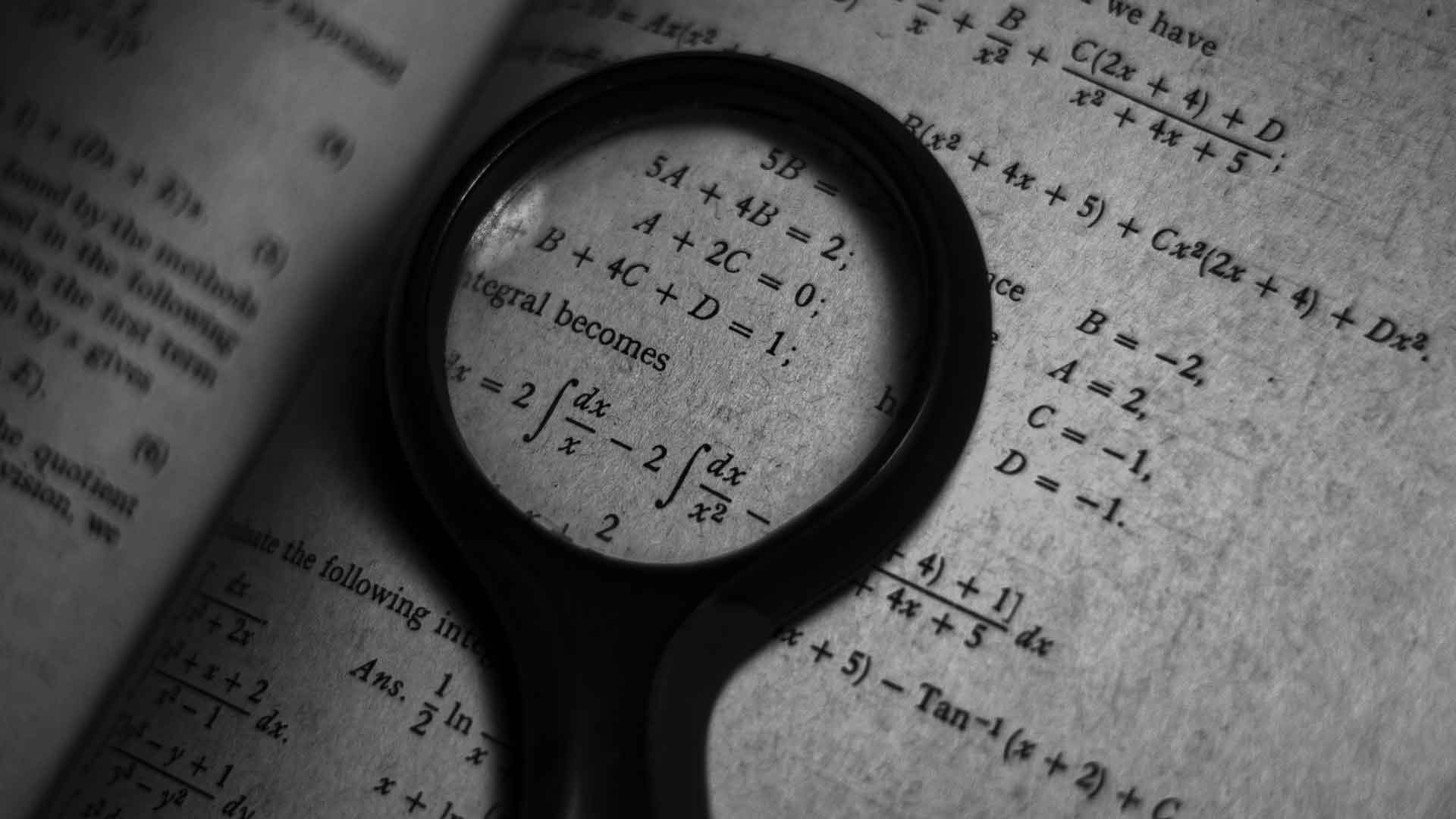### What are Actuarial Mathematics?

Actuarial science refers to the unique blend of several different fields of study; it serves the purpose of providing quantifiable guidelines for business decisions involving risk evaluation. The mathematics required by this science is a complex blend of calculus, statistics, financial math, and numerical modeling.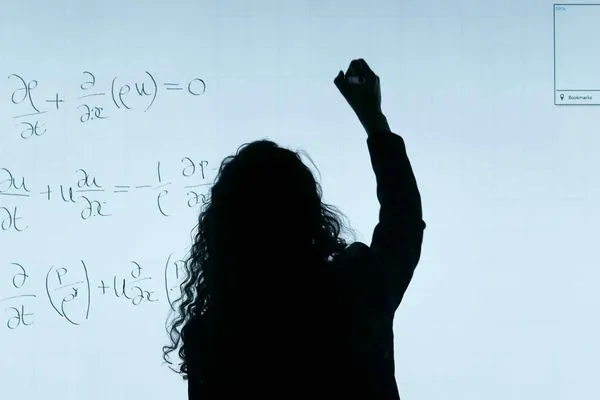### What are Mathematicians?

Mathematicians are people who specialize in the study of mathematics, the manipulation of numbers, variables, and abstract concepts in equations and theorems. They can work on a variety of topics, from the history of math to theoretical mathematics, and they work in many industries, including computer science and engineering.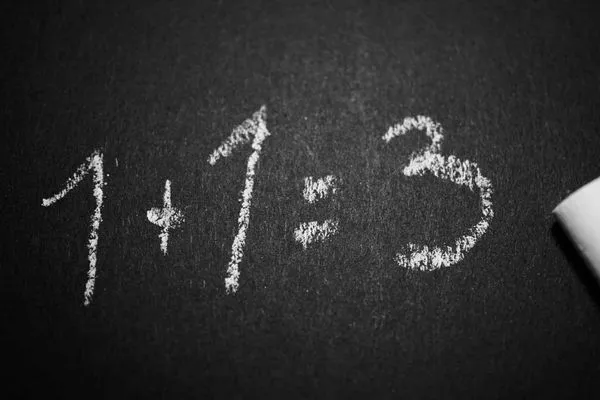### What Are the Best Tips to Improve Math Skills?

For many people, math can be a challenge in school and in adult life. There are some ways to improve math skills, however, that can make this subject less daunting. First, when doing math homework or other assignments, it is important to take the time to work through each problem and try to understand the larger concepts behind it.### What is a Quadratic Equation?

A quadratic equation consists of a single variable with three terms in the standard form: ax2 + bx + c = 0. The first quadratic equations were developed as a method used by Babylonian mathematicians around 2000 BC to solve simultaneous equations. Quadratic equations can be applied to problems in physics involving parabolic motion, path, shape, and stability. Several methods have evolved to simplify the solution of such equations for the variable x.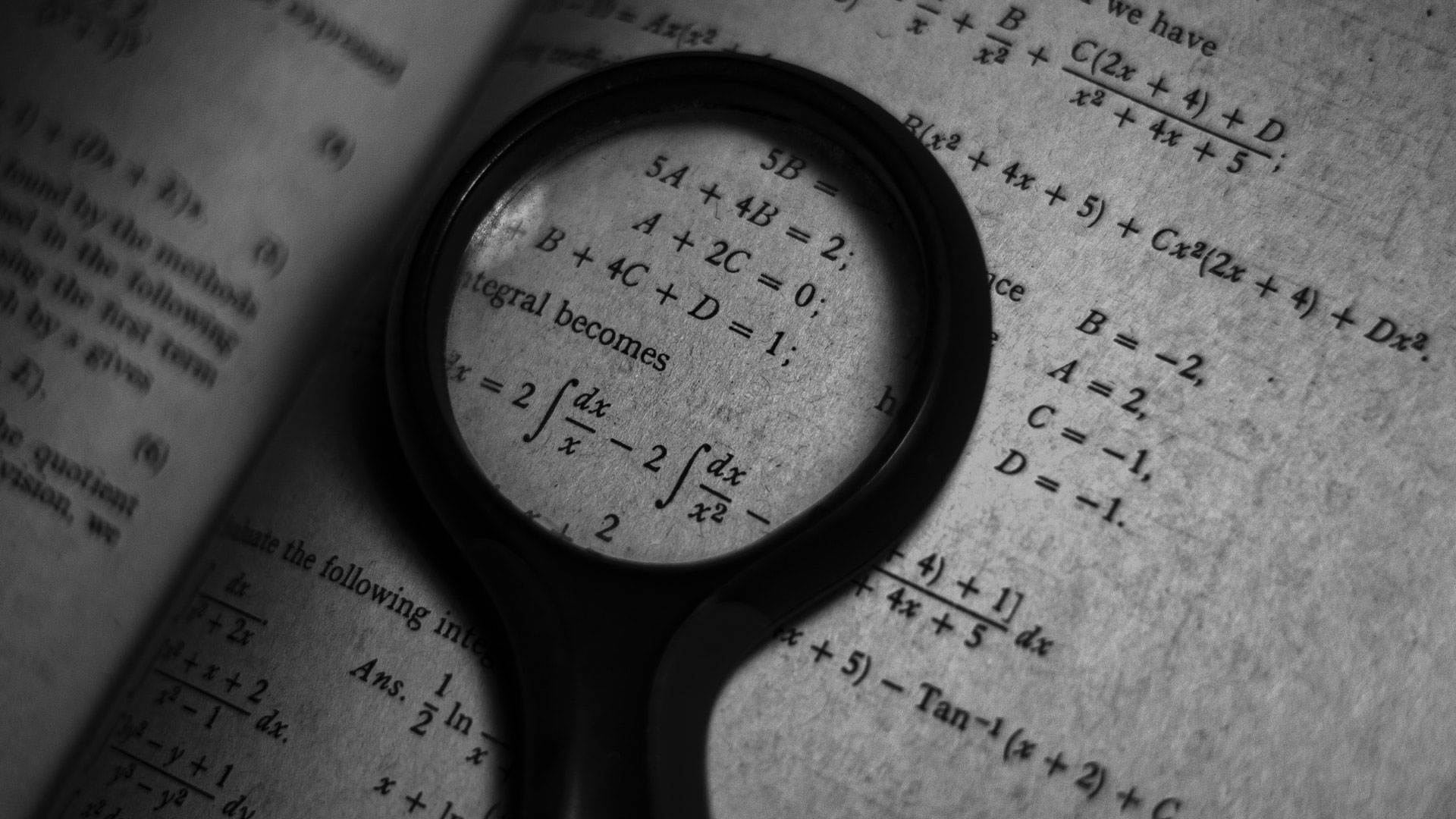### What are the Different Disciplines of Mathematics?

Mathematics includes many disciplines that have evolved throughout history. Simple examples include addition and subtraction, while extremely complex forms include chaos and games theories. Traditionally, however, the high school and early college years focus on the following disciplines.### In Mathematics, What Are Parameters?

Parameters are a special type of mathematical variable. A parametric equation contains one or more parametric variables that have multiple possible values. The value of each parameter is held constant when the function is used. In the statistical branches of mathematics, a parameter is an estimated numerical value for a population characteristic.### What Are the Different Types of Tutor Qualifications?

Tutor qualifications may include an undergraduate degree, a teaching credential or a person may simply work as a private tutor after demonstrating proficiency in a particular subject. While possessing professional qualifications is often necessary for a person to become a tutor in certain settings, precise qualifications vary according to the tutoring job being offered.### What is a Math Refresher?

A math refresher is an informal term that applies to a situation where a student may need remedial math work. Often, the student may take advantage of such courses during the course of a normal school day as an elective, and receive some credit for it.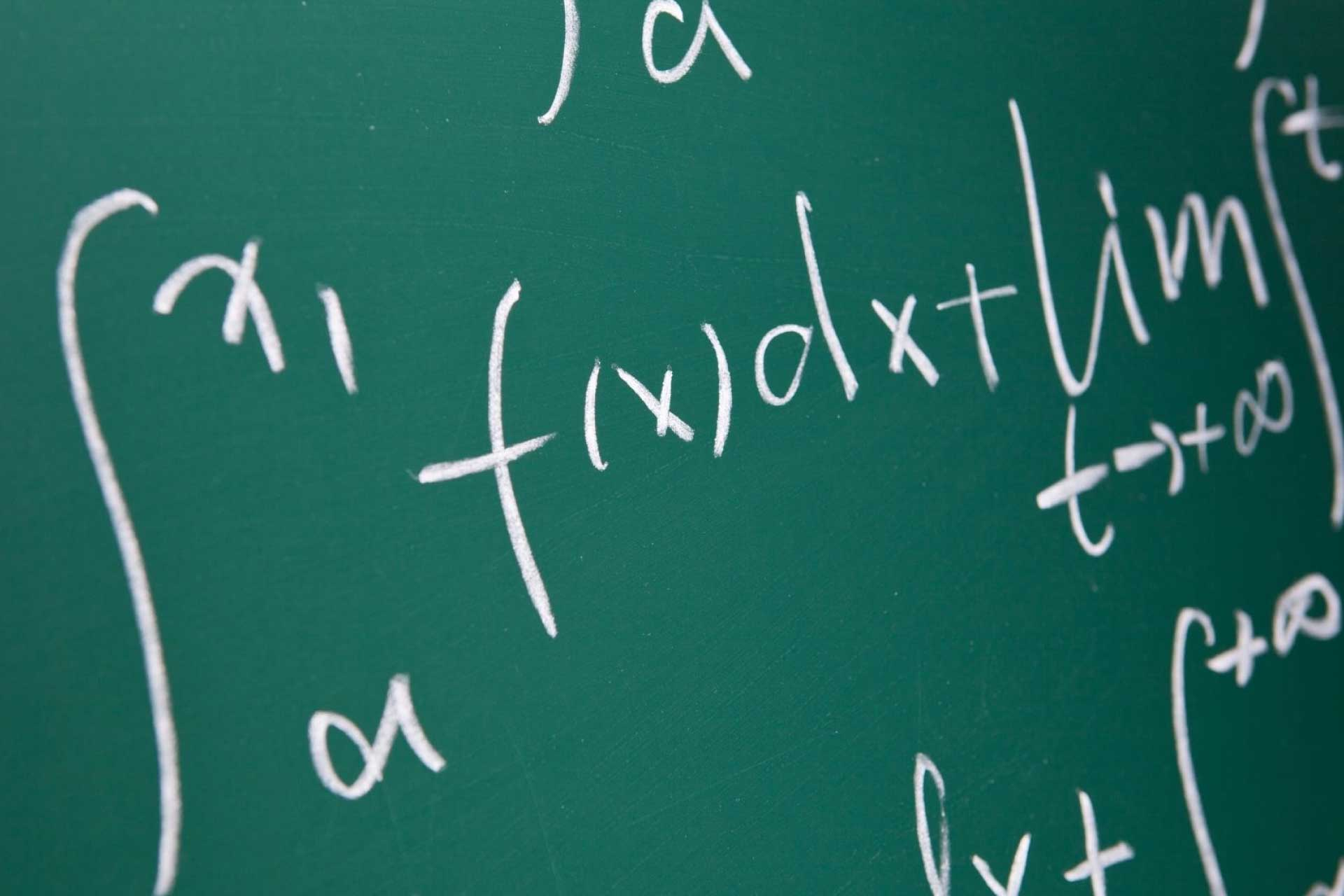### What is Calculus? Formula, Definition & Applications

The branch of mathematics called calculus originates from describing the basic physical properties of our universe, such as the motion of planets, and molecules. Calculus approaches the paths of objects in motion as curves, or functions, and then determines the value of these functions to calculate their rate of change, area, or volume.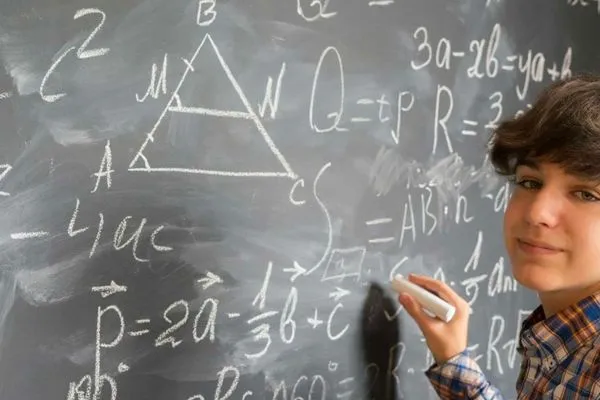### What Is Accelerated Math®?

Accelerated Math® most commonly refers to a computer-based mathematics learning system for pre-kindergarten through 12th grade, which is made by a company called Renaissance Learning. The computer software delivers customized, individualized math assignments to each student, which allows each child to move at his or her own pace.### What Is a Complex Conjugate In Mathematics?

In mathematics, a complex conjugate is a pair of two-component numbers called complex numbers. Each of these complex numbers possesses a real number component added to an imaginary component. Though their value is equal, the sign of one of the imaginary components in the pair of complex conjugate numbers is opposite to the sign of the other.### What Are the Best Tips for Learning Math?

The majority of experts agree that the best way of learning math is constant practice; although some people might have a natural aptitude for mathematics, high-level skill in math can be developed in most people. Individuals who want to perform better in math can use a wide variety of study and practice aids to hone their abilities, such as worksheets and online classes.### What is Biostatistics?

Biostatistics is, in effect, two words — and two fields of study — combined. The bio part involves biology, the study of living things. The statistics part involves the accumulation, tracking, analysis, and application of data. Biostatistics is the use of statistics procedures and analysis in the study and practice of biology. As such, it has many real-world and scientific applications.### What is Mathematics Disorder?

A mathematics disorder is a learning disorder in which a person, often a child, will have problems learning math skills and using them in everyday life. This disorder sometimes goes hand in hand with another learning disorder like dyslexia or dysgraphia, but other times an individual may be considered average or even above average in other areas.### How Can I Find a Good Tutor for My Child?

There are many ways to find a qualified private tutor for your child. One of the most common ways to find a tutor is through recommendations and referrals. Many individuals ask family members and friends for recommendations of tutors they’ve used or considered using.### What Are the Best Tips for Teaching Children Mathematics?

There is no underestimating the importance of teaching children mathematics. Children who are able to understand the basic mathematical concepts are much more likely to excel in school. Teaching children mathematics is a progressive process. One should start by teaching the child the basics of addition, followed by subtraction and multiplication, then ending with division, which is often the hardest mathematical concept for a child to learn.